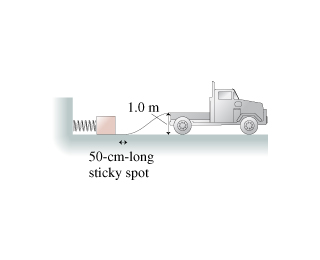# Problem: A freight company uses a compressed spring to shoot 1.90-kg packages up a 1.00-m-high frictionless ramp into a truck, as the figure (Figure 1) shows. The spring constant is 378 N/m and the spring is compressed 33.0 cm.What is the speed of the package when it reaches the truck?

###### FREE Expert Solution

Since there are no non-conservative forces acting, the initial potential energy of the spring is equal to the sum of final potential and kinetic energies of the truck.

$\begin{array}{rcl}{\mathbf{E}}_{\mathbf{p}\mathbf{.}\mathbf{s}}& \mathbf{=}& \mathbf{P}{\mathbf{E}}_{\mathbf{f}}\mathbf{+}\mathbf{K}{\mathbf{E}}_{\mathbf{f}}\\ & \mathbf{=}& \mathbf{m}\mathbf{g}\mathbf{h}\mathbf{+}\frac{\mathbf{1}}{\mathbf{2}}\mathbf{m}{\mathbf{v}}^{\mathbf{2}}\\ \frac{\mathbf{1}}{\mathbf{2}}\mathbf{m}{\mathbf{v}}^{\mathbf{2}}& \mathbf{=}& {\mathbf{E}}_{\mathbf{p}\mathbf{.}\mathbf{s}}\mathbf{-}\mathbf{m}\mathbf{g}\mathbf{h}\\ \mathbf{v}& \mathbf{=}& \sqrt{\frac{\mathbf{2}\mathbf{\left(}{\mathbf{E}}_{\mathbf{p}\mathbf{.}\mathbf{s}}\mathbf{-}\mathbf{m}\mathbf{g}\mathbf{h}\mathbf{\right)}}{\mathbf{m}}}\\ & \mathbf{=}& \sqrt{\frac{\mathbf{2}\mathbf{\left(}{\mathbf{E}}_{\mathbf{p}\mathbf{.}\mathbf{s}}\mathbf{-}\mathbf{m}\mathbf{g}\mathbf{h}\mathbf{\right)}}{\mathbf{m}}}\end{array}$

98% (27 ratings)###### Problem DetailsA freight company uses a compressed spring to shoot 1.90-kg packages up a 1.00-m-high frictionless ramp into a truck, as the figure (Figure 1) shows. The spring constant is 378 N/m and the spring is compressed 33.0 cm.

What is the speed of the package when it reaches the truck?